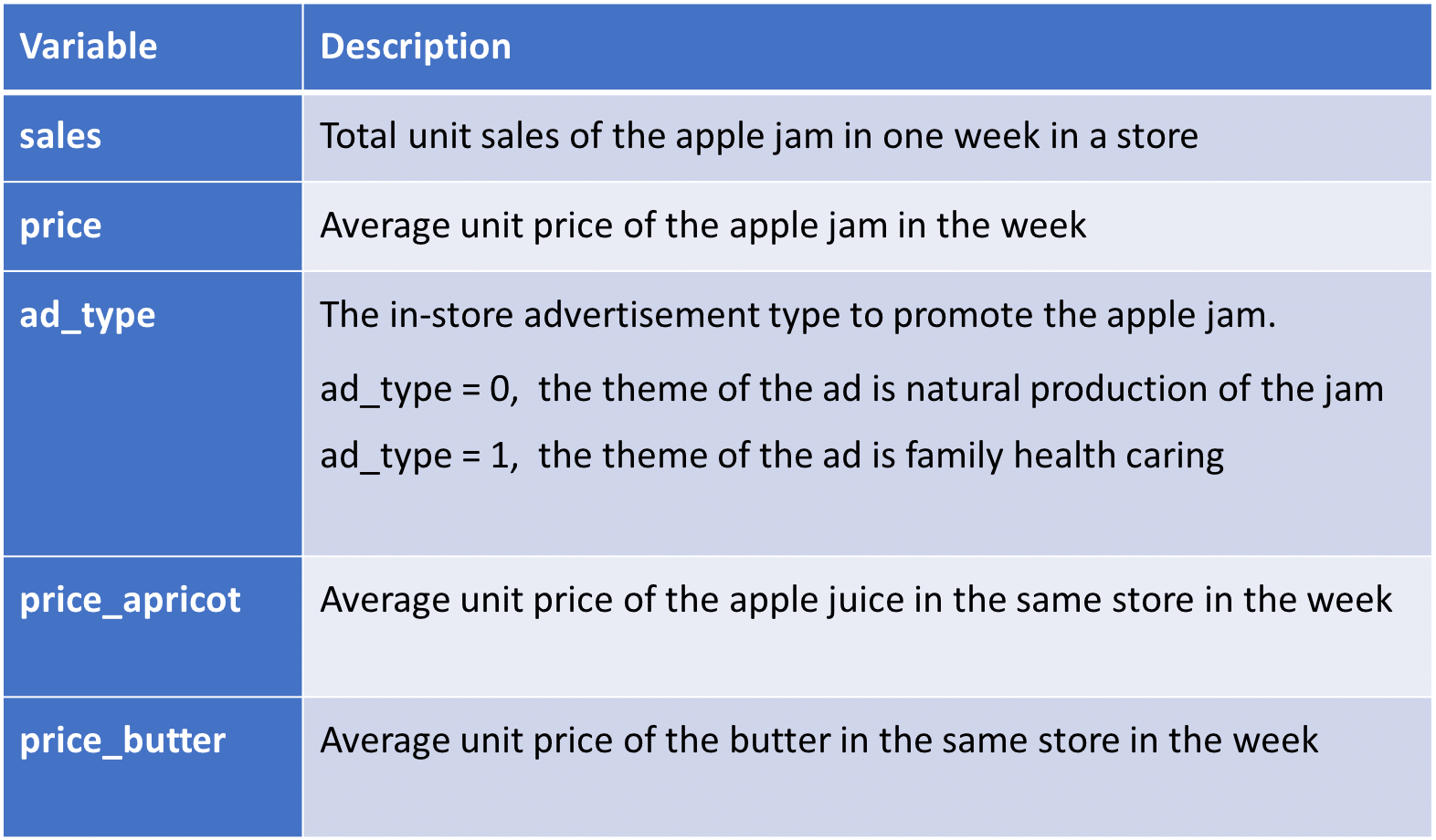# Ad Effectiveness and Price Elasticity Analysis by Example

• Home
• Article
• Ad Effectiveness and Price Elasticity Analysis by ExampleXYZ is selling a new apple jam in some of its stores for pilot testing. The marketing team of XYZ wants to analyze ad effectiveness by measuring the following:

1. Which type of in-store advertisement is more effective? They have placed two types of ads in stores for testing, one theme is a natural production of the jam, the other theme is family health caring;
2. The Price Elasticity – the reactions of sales quantity of the apple jam to its price change;
3. The Cross-price Elasticity – the reactions of sales quantity of the apple jam to the price changes of other products such as apricot jam and butter in the same store;
4. How to find the best unit price of the apple jam can maximize the profit and forecast sales with that price.

The marketing team randomly sampled 30 observations and constructed the following dataset for the analysis. There are 5 variables (data columns) in the dataset.

###The marketing team wants to find out the best ad effectiveness for sales between the two types of ads, one is with a natural production theme; the other is with a family health caring theme. So they can place the better one into all of XYZ’s stores after the pilot period.

To find out the better ad, we can calculate and compare the mean of sales with the two different ad types at the first step. One way to do that is to subset the data using R into two datasets, one with ad_type == 0 and the other with ad_type == 0.

The mean of sales with nature product theme is about 190; the mean of sales with family health caring theme is about 250. It looks like that the latter one is better. However, this is only the conclusion based on the sample with only 30 observations randomly selected. To find out how likely the conclusion is correct for the whole population, it is necessary to do statistical testing – a two-sample t-test.

It is important to check the assumptions of t-tests, which assume the observations are normally distributed and independent, before conducting the t-tests. Otherwise, the results of t-tests are not valid. The observations are independent since they were randomly sampled. We check the normality by plotting the distribution shapes of the two groups of sales data using box-plot and density distribution. We can also apply Shapiro-Wilk tests to check the normality assumption. The p-value in our example of the Shapiro-Wilk is less than 0.05, so there is no strong evidence to reject the null hypothesis that the two groups of sales data are normally distributed.

The result of the p-value of the t-test is less than 0.05. We have strong evidence to say that the population means of the sales with the two different ad types are different because the p-value of the t-test is very small;

So the conclusion is that the option with the theme of family health caring results in better ad effectiveness.

Sales Drivers and Price Elasticity Analysis

With the information given in the data set, we can explore how apple jam price, ad type, apricot jam price, butter price influence the sales of apple jam in a store by multiple linear regression analysis. Here, “sales” is the dependent variable and the others are independent variables.

Based on the multiple regression model, we can accept the regression result and construct the formula of sales as follows:

Sales = 774.81 – 51.24 * price + 29.74 * ad_type + 22.1 * price_apricot – 25.28 * price_butter

With the model established, we can analyze the Price Elasticity(PE) and Cross-price Elasticity(CPE) to predict the reactions of sales quantity to price. “Price elasticity is defined as %ΔQ/%ΔP, which indicates the percent change in quantity divided by the percent change in price; Cross-price Elasticity is the percent change in quantity divided by the change in the price of some other product.”

• PE = (ΔQ/Q) / (ΔP/P) = (ΔQ/ΔP) * (P/Q) = -51.24 * 0.045 = -2.3
• P is price, Q is sales quantity
• ΔQ/ΔP = -51.24, the parameter before the variable “price” in the above model
• P/Q = 9.738 / 216.7 = 0.045, P is the mean of prices in the dataset, so does Q
• The PE indicates that a 10% decrease in price will increase the sales by 23%, and vice versa.

We can further calculate the CPE on apricot jam and butter to analyze how the change of apricot jam and cookies price influences apple jam sales.

### Optimal Pricing and Sales Prediction

Usually, companies want to get higher profits rather than just higher sales quantity. So, how to set the optimal price for the new apple jam to get the maximum profit based on the dataset collected in the pilot period and the regression model above?

To simplify the question, we can let the ad_type = 1, the price_apricot = 7.659 (mean value), and the price_jam = 9.738 (mean value).

The regression model is simplified as follows:-

• Sales = 774.81 – 51.24 * price + 29.74 * 1 + 22.1 * 7.659 – 25.28 * 9.738
• Sales = 772.64 – 51.24*price

Assume the marginal cost(C) per unit of apple jam is 5. We can calculate the profit (Y) by the following formula.

• Y = (price – C) * Sales Quantity = (price – 5) * (772.64 – 51.24*price)
• Y = – 51.24 * price^2 + 1028.84 * price – 3863.2

To get the optimal price to maximize Y, we can use optimize function in R to maximize the objective function (Y). The optimize function will calculate the optimal price of the apple jam. We can further use the model to predict the sales using the optimal price.